# AP_Physics_1_-_Ch_5_Work_and_Energy.ppt

28 May 2023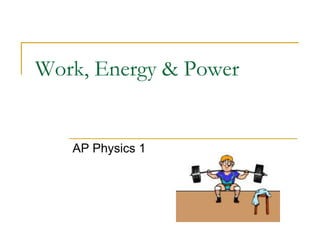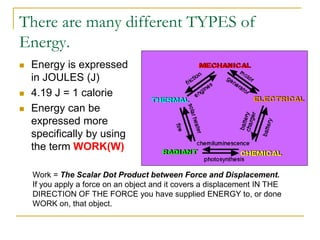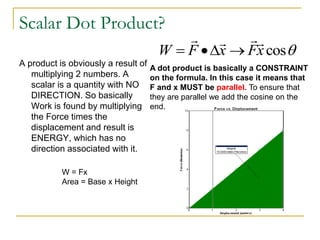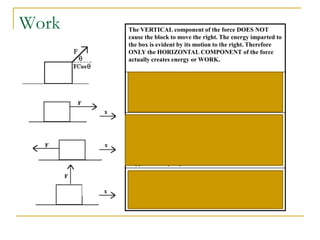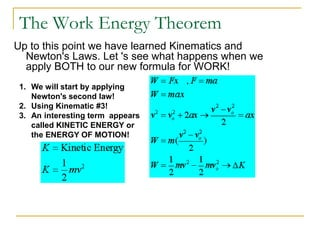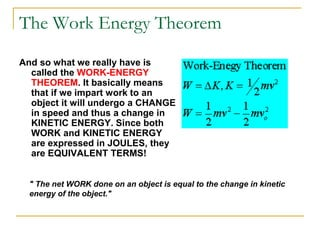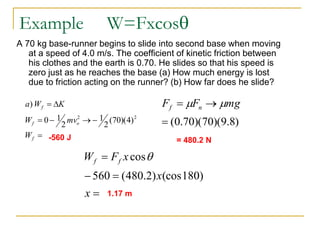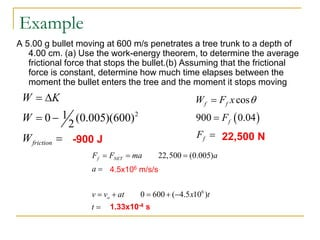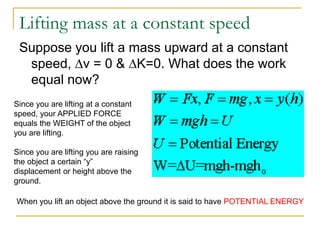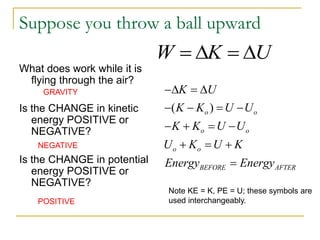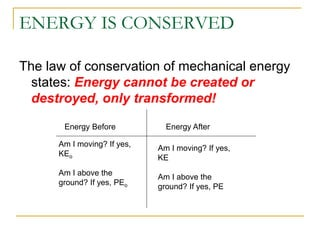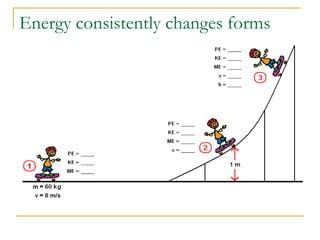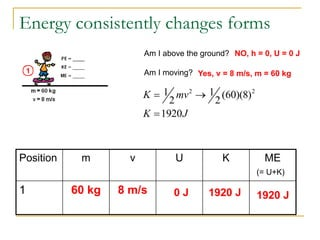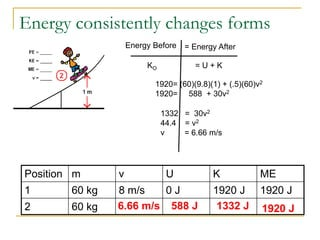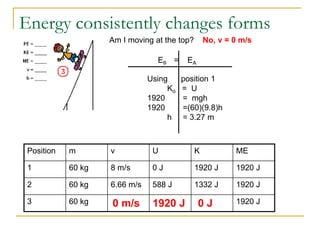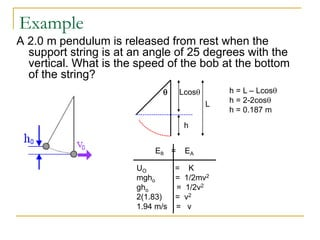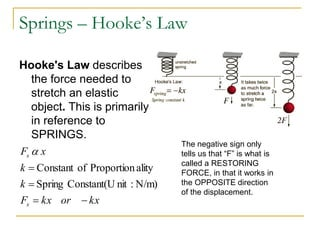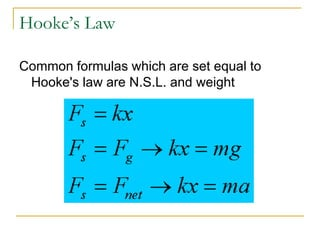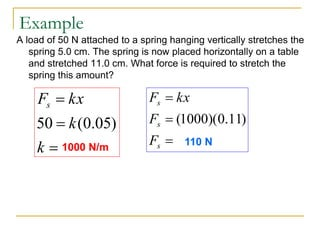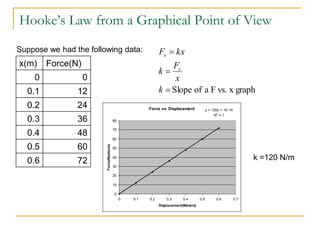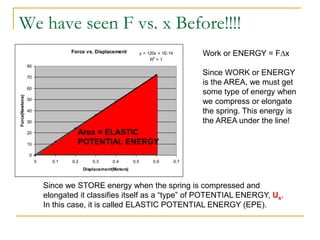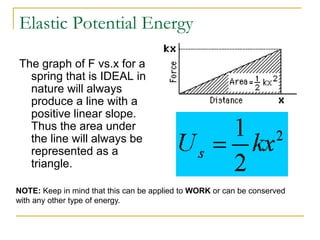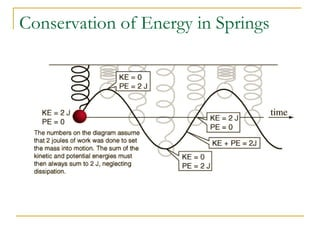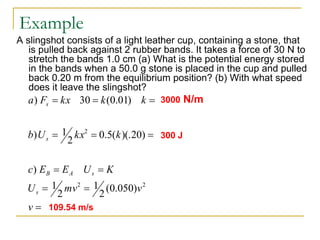1 sur 25

### AP_Physics_1_-_Ch_5_Work_and_Energy.ppt

• 1. Work, Energy & Power AP Physics 1
• 2. There are many different TYPES of Energy.  Energy is expressed in JOULES (J)  4.19 J = 1 calorie  Energy can be expressed more specifically by using the term WORK(W) Work = The Scalar Dot Product between Force and Displacement. If you apply a force on an object and it covers a displacement IN THE DIRECTION OF THE FORCE you have supplied ENERGY to, or done WORK on, that object.
• 3. Scalar Dot Product? A product is obviously a result of multiplying 2 numbers. A scalar is a quantity with NO DIRECTION. So basically Work is found by multiplying the Force times the displacement and result is ENERGY, which has no direction associated with it.  cos x F x F W         A dot product is basically a CONSTRAINT on the formula. In this case it means that F and x MUST be parallel. To ensure that they are parallel we add the cosine on the end. W = Fx Area = Base x Height
• 4. Work The VERTICAL component of the force DOES NOT cause the block to move the right. The energy imparted to the box is evident by its motion to the right. Therefore ONLY the HORIZONTAL COMPONENT of the force actually creates energy or WORK. When the FORCE and DISPLACEMENT are in the SAME DIRECTION you get a POSITIVE WORK VALUE. The ANGLE between the force and displacement is ZERO degrees. What happens when you put this in for the COSINE? When the FORCE and DISPLACEMENT are in the OPPOSITE direction, yet still on the same axis, you get a NEGATIVE WORK VALUE. This negative doesn't mean the direction!!!! IT simply means that the force and displacement oppose each other. The ANGLE between the force and displacement in this case is 180 degrees. What happens when you put this in for the COSINE? When the FORCE and DISPLACEMENT are PERPENDICULAR, you get NO WORK!!! The ANGLE between the force and displacement in this case is 90 degrees. What happens when you put this in for the COSINE?
• 5. The Work Energy Theorem Up to this point we have learned Kinematics and Newton's Laws. Let 's see what happens when we apply BOTH to our new formula for WORK! 1. We will start by applying Newton's second law! 2. Using Kinematic #3! 3. An interesting term appears called KINETIC ENERGY or the ENERGY OF MOTION!
• 6. The Work Energy Theorem And so what we really have is called the WORK-ENERGY THEOREM. It basically means that if we impart work to an object it will undergo a CHANGE in speed and thus a change in KINETIC ENERGY. Since both WORK and KINETIC ENERGY are expressed in JOULES, they are EQUIVALENT TERMS! " The net WORK done on an object is equal to the change in kinetic energy of the object."
• 7. Example W=Fxcos A 70 kg base-runner begins to slide into second base when moving at a speed of 4.0 m/s. The coefficient of kinetic friction between his clothes and the earth is 0.70. He slides so that his speed is zero just as he reaches the base (a) How much energy is lost due to friction acting on the runner? (b) How far does he slide? ) 8 . 9 )( 70 )( 70 . 0 (    mg F F n f   = 480.2 N        f o f f W mv W K W a 2 2 ) 4 )( 70 ( 2 1 2 1 0 ) -560 J     x x x F W f f ) 180 (cos ) 2 . 480 ( 560 cos 1.17 m
• 8. Example A 5.00 g bullet moving at 600 m/s penetrates a tree trunk to a depth of 4.00 cm. (a) Use the work-energy theorem, to determine the average frictional force that stops the bullet.(b) Assuming that the frictional force is constant, determine how much time elapses between the moment the bullet enters the tree and the moment it stops moving 2 1 0 (0.005)(600) 2 friction W K W W      -900 J   cos 900 0.04 f f f f W F x F F     22,500 N 6 22,500 (0.005) 0 600 ( 4.5 10 ) f NET o F F ma a a v v at x t t           4.5x106 m/s/s 1.33x10-4 s
• 9. Lifting mass at a constant speed Suppose you lift a mass upward at a constant speed, v = 0 & K=0. What does the work equal now? Since you are lifting at a constant speed, your APPLIED FORCE equals the WEIGHT of the object you are lifting. Since you are lifting you are raising the object a certain “y” displacement or height above the ground. When you lift an object above the ground it is said to have POTENTIAL ENERGY
• 10. Suppose you throw a ball upward What does work while it is flying through the air? Is the CHANGE in kinetic energy POSITIVE or NEGATIVE? Is the CHANGE in potential energy POSITIVE or NEGATIVE? W K U     GRAVITY NEGATIVE POSITIVE ( ) o o o o o o BEFORE AFTER K U K K U U K K U U U K U K Energy Energy                Note KE = K, PE = U; these symbols are used interchangeably.
• 11. ENERGY IS CONSERVED The law of conservation of mechanical energy states: Energy cannot be created or destroyed, only transformed! Energy Before Energy After Am I moving? If yes, KEo Am I above the ground? If yes, PEo Am I moving? If yes, KE Am I above the ground? If yes, PE
• 12. Energy consistently changes forms
• 13. Energy consistently changes forms Position m v U K ME 1 60 kg 8 m/s Am I above the ground? Am I moving? NO, h = 0, U = 0 J 0 J Yes, v = 8 m/s, m = 60 kg 2 2 1 1 (60)(8) 2 2 1920 K mv K J    1920 J (= U+K) 1920 J
• 14. Energy consistently changes forms Position m v U K ME 1 60 kg 8 m/s 0 J 1920 J 1920 J 2 60 kg Energy Before = Energy After KO = U + K 1920= (60)(9.8)(1) + (.5)(60)v2 1920= 588 + 30v2 588 J 1332 = 30v2 44.4 = v2 v = 6.66 m/s 6.66 m/s 1920 J 1332 J
• 15. Energy consistently changes forms Position m v U K ME 1 60 kg 8 m/s 0 J 1920 J 1920 J 2 60 kg 6.66 m/s 588 J 1332 J 1920 J 3 60 kg 1920 J Am I moving at the top? No, v = 0 m/s 0 m/s 0 J 1920 J EB = EA Using position 1 Ko = U 1920 = mgh 1920 =(60)(9.8)h h = 3.27 m
• 16. Example A 2.0 m pendulum is released from rest when the support string is at an angle of 25 degrees with the vertical. What is the speed of the bob at the bottom of the string? L  Lcos h h = L – Lcos h = 2-2cos h = 0.187 m EB = EA UO = K mgho = 1/2mv2 gho = 1/2v2 2(1.83) = v2 1.94 m/s = v
• 17. Springs – Hooke’s Law Hooke's Law describes the force needed to stretch an elastic object. This is primarily in reference to SPRINGS. kx or kx F k k x F s s     N/m) : nit Constant(U Spring ality Proportion of Constant  The negative sign only tells us that “F” is what is called a RESTORING FORCE, in that it works in the OPPOSITE direction of the displacement.
• 18. Hooke’s Law Common formulas which are set equal to Hooke's law are N.S.L. and weight
• 19. Example A load of 50 N attached to a spring hanging vertically stretches the spring 5.0 cm. The spring is now placed horizontally on a table and stretched 11.0 cm. What force is required to stretch the spring this amount?    k k kx Fs ) 05 . 0 ( 50 1000 N/m    s s s F F kx F ) 11 . 0 )( 1000 ( 110 N
• 20. Hooke’s Law from a Graphical Point of View x(m) Force(N) 0 0 0.1 12 0.2 24 0.3 36 0.4 48 0.5 60 0.6 72 graph x vs. F a of Slope    k x F k kx F s s Suppose we had the following data: Force vs. Displacement y = 120x + 1E-14 R2 = 1 0 10 20 30 40 50 60 70 80 0 0.1 0.2 0.3 0.4 0.5 0.6 0.7 Displacement(Meters) Force(Newtons) k =120 N/m
• 21. We have seen F vs. x Before!!!! Force vs. Displacement y = 120x + 1E-14 R2 = 1 0 10 20 30 40 50 60 70 80 0 0.1 0.2 0.3 0.4 0.5 0.6 0.7 Displacement(Meters) Force(Newtons) Work or ENERGY = Fx Since WORK or ENERGY is the AREA, we must get some type of energy when we compress or elongate the spring. This energy is the AREA under the line! Area = ELASTIC POTENTIAL ENERGY Since we STORE energy when the spring is compressed and elongated it classifies itself as a “type” of POTENTIAL ENERGY, Us. In this case, it is called ELASTIC POTENTIAL ENERGY (EPE).
• 22. Elastic Potential Energy The graph of F vs.x for a spring that is IDEAL in nature will always produce a line with a positive linear slope. Thus the area under the line will always be represented as a triangle. NOTE: Keep in mind that this can be applied to WORK or can be conserved with any other type of energy.
• 23. Conservation of Energy in Springs
• 24. Example A slingshot consists of a light leather cup, containing a stone, that is pulled back against 2 rubber bands. It takes a force of 30 N to stretch the bands 1.0 cm (a) What is the potential energy stored in the bands when a 50.0 g stone is placed in the cup and pulled back 0.20 m from the equilibrium position? (b) With what speed does it leave the slingshot?            v v mv U K U E E c k kx U b k k kx F a s s A B s s 2 2 2 ) 050 . 0 ( 2 1 2 1 ) ) 20 )(. ( 5 . 0 2 1 ) ) 01 . 0 ( 30 ) 3000 N/m 300 J 109.54 m/s
• 25. Power One useful application of Energy is to determine the RATE at which we store or use it. We call this application POWER! As we use this new application, we have to keep in mind all the different kinds of substitutions we can make. Unit = WATT or Horsepower